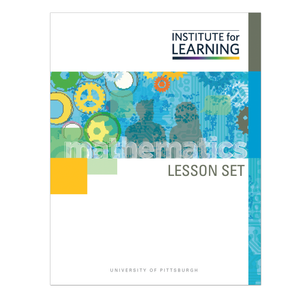# Decimal Operations: Multiplication and Division (Grade 5)

Regular price \$6.25 \$0.00 Unit price per

This lesson set provides an introduction to decimal multiplication and division problems in which students have to consider how many groups of a fixed size comprise a total. Students will:

• apply understanding of division with whole numbers to division involving numbers less than one;
• recognize the meaning of the dividend and the divisor and what happens when a unit fraction is in either position (but not both); and
• use a variety of representations to respond to a problem situation and weigh the advantages and disadvantages of such representations.

Standards: 5.NBT.B.5, 5.NBT.B.7 (Standard 5.NBT.B.6 is indirectly addressed.)